## Hypothesis Testing

When facing a hypothesis test problem there are steps to take to earn full credit. Similar to confidence intervals, there are hypothesis test for various population parameters with each using a different formula. Fortunately, most intro stat classes will either provide a formula sheet or allow you to create one. Here are the most common hypothesis test that are covered.

Hypothesis TestTest Statistic

One Sample t Test for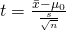Paired Sample t Test for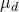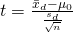Two Sample t Test for(unpooled)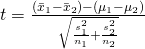Two Sample t Test for(pooled)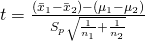One Proportion z Test for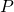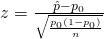Two Proportion z Test for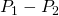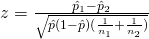### Steps to a Hypothesis Test

1. Null and Alternative Hypothesis
The first step is to figure out what the null and alternative hypothesis are. The null hypothesis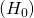will have the parameter be set equal to some number that will be found in the context of the problem. The alternative hypothesis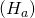will have the parameter with some inequality, either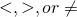. If the alternative hypothesis is(less than) or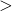(greater than), then the test will be one sided. If the alternative hypothesis is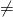, then the test will be two sided.
2. Calculate the Test Statistic
This part will depend on the hypothesis being performed. We can use the table above to figure out what formula is needed. We can then get each variable from the context of the problem. After we identify each variable in our formula, we calculate the test statistic.
3. Find the P-Value
The p-value will be either given to you in the problem or will have to be found using some program. In a homework, the p-value can be calculated using one of the following programs: JMP, Excel, or a TI-84 calculator. In an exam, they will likely give you a screenshot of output from the given programs.
4. Conclusion
There are two conclusion we can make depending on the p-value. To decide, we compare our p-value to the level of significance (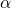). If the p-value is less than our significance level (usually .05), we reject the null hypothesis. If the p-value is greater than our significance level, we fail to reject (FTR) the null hypothesis.